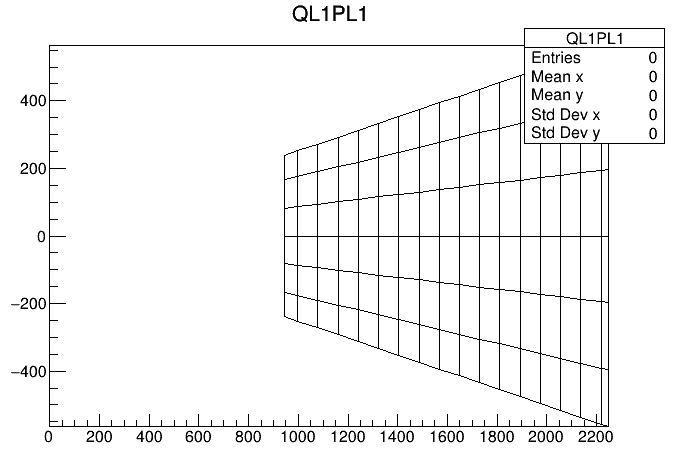# Filling TH2Poly by bin number/name

Dear ROOTers,

I have the following code:

``````TH2Poly *h2p = new TH2Poly();
h2p->SetName("h2poly_name");
h2p->SetTitle("h2poly_title");
double px[] = {x1, x2, x3, x4};
double py[] = {y1, y2, y3, y4};
for (int i=0; i<100; i++) {
h2p->Fill(1400, 0);
}
h2p->Draw();
h2p->Write();
``````

This works.
However, I would like to fill my `TH2Poly` not by x, y coords. ((1400, 0) in the above example) but rather by bin number or rather bin name. The class reference for `TH2Poly` states it can be done by name but doesn’t provide an option to `AddBin` by bin name in addition to x,y coords. Nevertheless, I see that BinNumber can be extracted from `TH2Poly` once they are written.

How can this be overcome? Ideally, I would be able to fill the `TH2Poly` by bin number or name as shown to work with the USA example. Alas, this doesn’t work for me.

Any advice would be highly appreciated.

Kind regards,

Roy

_ROOT Version: 6-18-04
_Platform: 5.4.0-81-generic #91-Ubuntu
_Compiler: C++ ROOT compiler

I think @couet will be able to help once he’s back next week

1 Like

Hi again to all,

Thanks @bellenot.

To be more specific following progress I’ve made, whenever @couet et al. see this, let me add:

I’ve taken a closer look at the USA/Europe examples and tried to implement with `TGraphs`, something like:

``````TH2Poly *h2p = new TH2Poly();
TGraph *gr = new TGraph();
while ( getline (pad_corners_file, line) ) {
double px[] = {x1, x2, x3, x4};
double py[] = {y1, y2, y3, y4};
gr->SetPoint(0, x1, y1);
gr->SetPoint(1, x2, y2);
gr->SetPoint(2, x3, y3);
gr->SetPoint(3, x4, y4);
//See two lines below; the first yields the desired outcome but the second is the one I wish to implement as it allows dealing with graphs (which can be associated with indices)
}
h2p->Draw();
``````

The first (`h2p->AddBin(4, px, py);`), second (`h2p->AddBin(gr);`) line yields the first, second figure (attached below), respectively, although one would expect to attain the exact same figure using the second line too. For some reason, with the second line, using `TGraphs` gives an outcome where all the graphs aren’t drawn expect the last (cf. bottom-right corner of the second figure).
What causes this? What have I missed?

FYI, whilst trying to debug I added to the loop lines like:

``````std::cout << "gr->GetX(), gr->GetY()  =   " << gr->GetX() << "   " << gr->GetY() << std::endl;
std::cout << "gr->GetX(), gr->GetY() =   " << gr->GetX() << "   " << gr->GetY() << std::endl;
``````

to verify the graphs store the correct information — indeed they do. Therefore I’m perplexed.

Any advice would be warmly welcomed!

Cheers,

RoyYes filling by name is possible. For instance in the “usa” example you can see:

``````  // Fill TH2Poly.
for (i=0; i<nx; i++) p->Fill(states[i], pop[i]);
``````

`states` is the name of the various USA states. simply a `char*`.

Of course you should name your graphs with `SetName`

2 Likes

This topic was automatically closed 14 days after the last reply. New replies are no longer allowed.